Publicité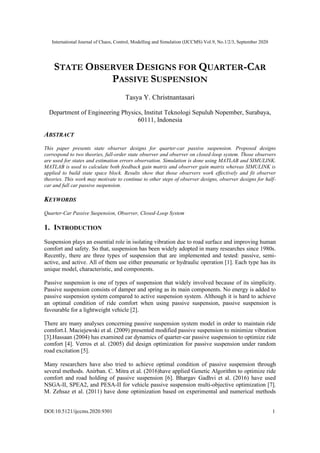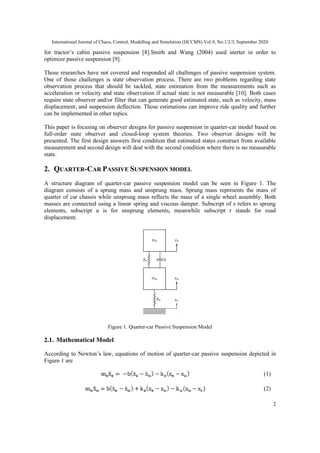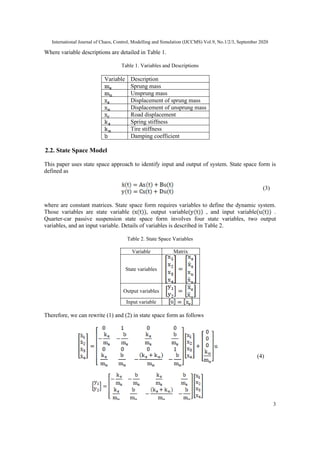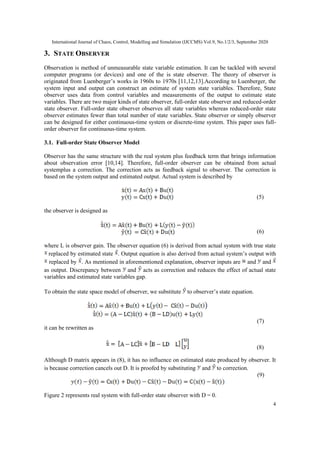Publicité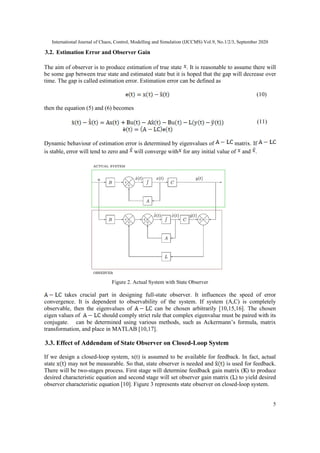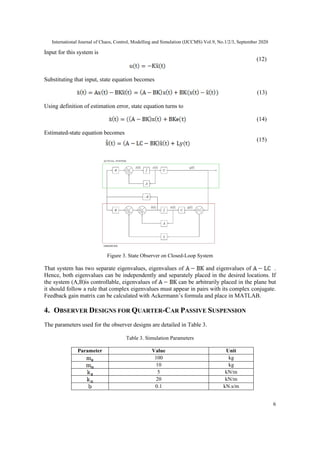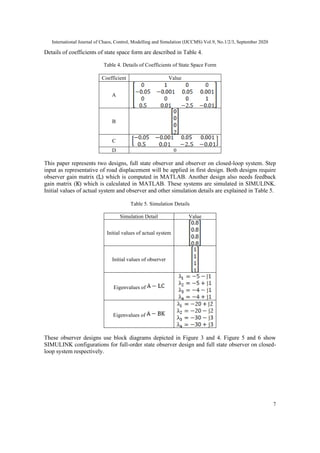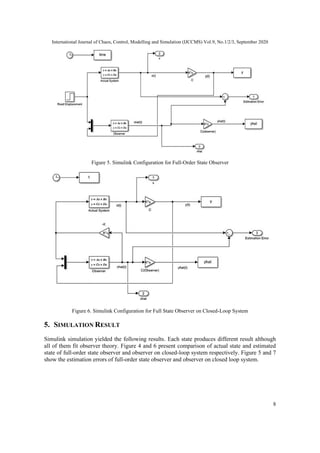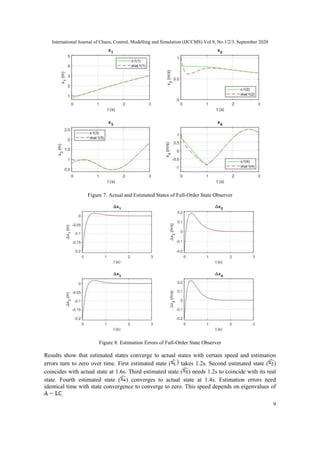Publicité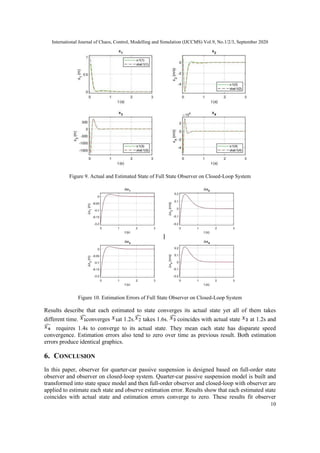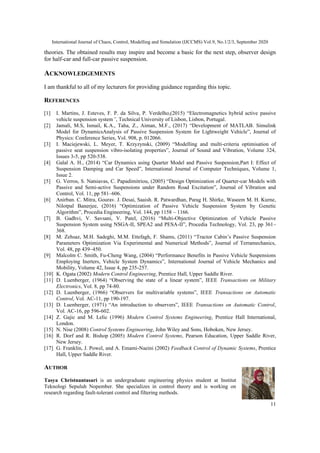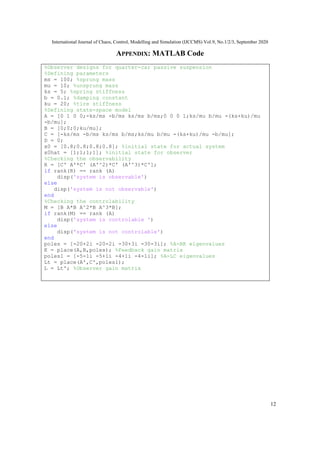Prochain SlideShare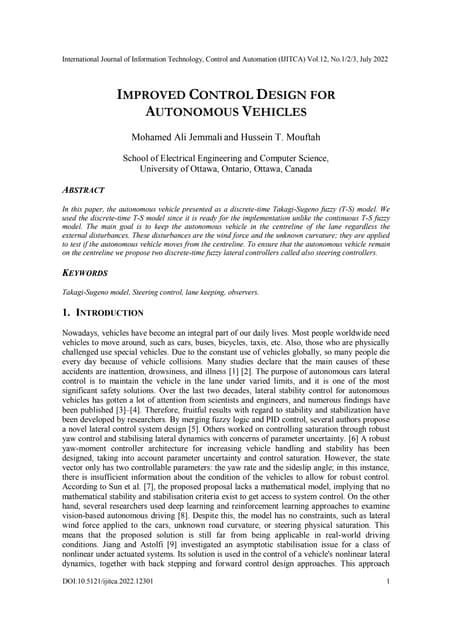IMPROVED CONTROL DESIGN FOR AUTONOMOUS VEHICLES
Chargement dans ... 3
1 sur 12
Publicité

### 9320ijccms01.pdf

1. International Journal of Chaos, Control, Modelling and Simulation (IJCCMS) Vol.9, No.1/2/3, September 2020 DOI:10.5121/ijccms.2020.9301 1 STATE OBSERVER DESIGNS FOR QUARTER-CAR PASSIVE SUSPENSION Tasya Y. Christnantasari Department of Engineering Physics, Institut Teknologi Sepuluh Nopember, Surabaya, 60111, Indonesia ABSTRACT This paper presents state observer designs for quarter-car passive suspension. Proposed designs correspond to two theories, full-order state observer and observer on closed-loop system. Those observers are used for states and estimation errors observation. Simulation is done using MATLAB and SIMULINK. MATLAB is used to calculate both feedback gain matrix and observer gain matrix whereas SIMULINK is applied to build state space block. Results show that those observers work effectively and fit observer theories. This work may motivate to continue to other steps of observer designs, observer designs for half- car and full car passive suspension. KEYWORDS Quarter-Car Passive Suspension, Observer, Closed-Loop System 1. INTRODUCTION Suspension plays an essential role in isolating vibration due to road surface and improving human comfort and safety. So that, suspension has been widely adopted in many researches since 1980s. Recently, there are three types of suspension that are implemented and tested: passive, semi- active, and active. All of them use either pneumatic or hydraulic operation . Each type has its unique model, characteristic, and components. Passive suspension is one of types of suspension that widely involved because of its simplicity. Passive suspension consists of damper and spring as its main components. No energy is added to passive suspension system compared to active suspension system. Although it is hard to achieve an optimal condition of ride comfort when using passive suspension, passive suspension is favourable for a lightweight vehicle . There are many analyses concerning passive suspension system model in order to maintain ride comfort.I. Maciejewski et al. (2009) presented modified passive suspension to minimize vibration .Hassaan (2004) has examined car dynamics of quarter-car passive suspension to optimize ride comfort . Verros et al. (2005) did design optimization for passive suspension under random road excitation . Many researchers have also tried to achieve optimal condition of passive suspension through several methods. Anirban. C. Mitra et al. (2016)have applied Genetic Algorithm to optimize ride comfort and road holding of passive suspension . Bhargav Gadhvi et al. (2016) have used NSGA-II, SPEA2, and PESA-II for vehicle passive suspension multi-objective optimization . M. Zehsaz et al. (2011) have done optimization based on experimental and numerical methods
2. International Journal of Chaos, Control, Modelling and Simulation (IJCCMS) Vol.9, No.1/2/3, September 2020 2 for tractor’s cabin passive suspension .Smith and Wang (2004) used inerter in order to optimize passive suspension . Those researches have not covered and responded all challenges of passive suspension system. One of those challenges is state observation process. There are two problems regarding state observation process that should be tackled, state estimation from the measurements such as acceleration or velocity and state observation if actual state is not measurable . Both cases require state observer and/or filter that can generate good estimated state, such as velocity, mass displacement, and suspension deflection. Those estimations can improve ride quality and further can be implemented in other topics. This paper is focusing on observer designs for passive suspension in quarter-car model based on full-order state observer and closed-loop system theories. Two observer designs will be presented. The first design answers first condition that estimated states construct from available measurement and second design will deal with the second condition where there is no measurable state. 2. QUARTER-CAR PASSIVE SUSPENSION MODEL A structure diagram of quarter-car passive suspension model can be seen in Figure 1. The diagram consists of a sprung mass and unsprung mass. Sprung mass represents the mass of quarter of car chassis while unsprung mass reflects the mass of a single wheel assembly. Both masses are connected using a linear spring and viscous damper. Subscript of s refers to sprung elements, subscript u is for unsprung elements, meanwhile subscript r stands for road displacement. Figure 1. Quarter-car Passive Suspension Model 2.1. Mathematical Model According to Newton’s law, equations of motion of quarter-car passive suspension depicted in Figure 1 are (1) (2)
3. International Journal of Chaos, Control, Modelling and Simulation (IJCCMS) Vol.9, No.1/2/3, September 2020 3 Where variable descriptions are detailed in Table 1. Table 1. Variables and Descriptions Variable Description Sprung mass Unsprung mass Displacement of sprung mass Displacement of unsprung mass Road displacement Spring stiffness Tire stiffness Damping coefficient 2.2. State Space Model This paper uses state space approach to identify input and output of system. State space form is defined as (3) where are constant matrices. State space form requires variables to define the dynamic system. Those variables are state variable ( , output variable , and input variable . Quarter-car passive suspension state space form involves four state variables, two output variables, and an input variable. Details of variables is described in Table 2. Table 2. State Space Variables Variable Matrix State variables Output variables Input variable Therefore, we can rewrite (1) and (2) in state space form as follows (4)
4. International Journal of Chaos, Control, Modelling and Simulation (IJCCMS) Vol.9, No.1/2/3, September 2020 4 3. STATE OBSERVER Observation is method of unmeasurable state variable estimation. It can be tackled with several computer programs (or devices) and one of the is state observer. The theory of observer is originated from Luenberger’s works in 1960s to 1970s [11,12,13].According to Luenberger, the system input and output can construct an estimate of system state variables. Therefore, State observer uses data from control variables and measurements of the output to estimate state variables. There are two major kinds of state observer, full-order state observer and reduced-order state observer. Full-order state observer observes all state variables whereas reduced-order state observer estimates fewer than total number of state variables. State observer or simply observer can be designed for either continuous-time system or discrete-time system. This paper uses full- order observer for continuous-time system. 3.1. Full-order State Observer Model Observer has the same structure with the real system plus feedback term that brings information about observation error [10,14]. Therefore, full-order observer can be obtained from actual systemplus a correction. The correction acts as feedback signal to observer. The correction is based on the system output and estimated output. Actual system is described by (5) the observer is designed as (6) where L is observer gain. The observer equation (6) is derived from actual system with true state replaced by estimated state . Output equation is also derived from actual system’s output with replaced by . As mentioned in aforementioned explanation, observer inputs are and and as output. Discrepancy between and acts as correction and reduces the effect of actual state variables and estimated state variables gap. To obtain the state space model of observer, we substitute to observer’s state equation. (7) it can be rewritten as (8) Although D matrix appears in (8), it has no influence on estimated state produced by observer. It is because correction cancels out D. It is proofed by substituting and to correction. (9) Figure 2 represents real system with full-order state observer with D = 0.
5. International Journal of Chaos, Control, Modelling and Simulation (IJCCMS) Vol.9, No.1/2/3, September 2020 5 3.2. Estimation Error and Observer Gain The aim of observer is to produce estimation of true state . It is reasonable to assume there will be some gap between true state and estimated state but it is hoped that the gap will decrease over time. The gap is called estimation error. Estimation error can be defined as (10) then the equation (5) and (6) becomes (11) Dynamic behaviour of estimation error is determined by eigenvalues of matrix. If is stable, error will tend to zero and will converge with for any initial value of and . Figure 2. Actual System with State Observer takes crucial part in designing full-state observer. It influences the speed of error convergence. It is dependent to observability of the system. If system (A,C) is completely observable, then the eigenvalues of can be chosen arbitrarily [10,15,16]. The chosen eigen values of should comply strict rule that complex eigenvalue must be paired with its conjugate. can be determined using various methods, such as Ackermann’s formula, matrix transformation, and place in MATLAB [10,17]. 3.3. Effect of Addendum of State Observer on Closed-Loop System If we design a closed-loop system, x(t) is assumed to be available for feedback. In fact, actual state may not be measurable. So that, state observer is needed and is used for feedback. There will be two-stages process. First stage will determine feedback gain matrix ( ) to produce desired characteristic equation and second stage will set observer gain matrix ( ) to yield desired observer characteristic equation . Figure 3 represents state observer on closed-loop system.
6. International Journal of Chaos, Control, Modelling and Simulation (IJCCMS) Vol.9, No.1/2/3, September 2020 6 Input for this system is (12) Substituting that input, state equation becomes (13) Using definition of estimation error, state equation turns to (14) Estimated-state equation becomes (15) Figure 3. State Observer on Closed-Loop System That system has two separate eigenvalues, eigenvalues of and eigenvalues of . Hence, both eigenvalues can be independently and separately placed in the desired locations. If the system (A,B)is controllable, eigenvalues of can be arbitrarily placed in the plane but it should follow a rule that complex eigenvalues must appear in pairs with its complex conjugate. Feedback gain matrix can be calculated with Ackermann’s formula and place in MATLAB. 4. OBSERVER DESIGNS FOR QUARTER-CAR PASSIVE SUSPENSION The parameters used for the observer designs are detailed in Table 3. Table 3. Simulation Parameters Parameter Value Unit 100 kg 10 kg 5 kN/m 20 kN/m 0.1 kN.s/m
7. International Journal of Chaos, Control, Modelling and Simulation (IJCCMS) Vol.9, No.1/2/3, September 2020 7 Details of coefficients of state space form are described in Table 4. Table 4. Details of Coefficients of State Space Form Coefficient Value A B C D 0 This paper represents two designs, full state observer and observer on closed-loop system. Step input as representative of road displacement will be applied in first design. Both designs require observer gain matrix ( ) which is computed in MATLAB. Another design also needs feedback gain matrix ( ) which is calculated in MATLAB. These systems are simulated in SIMULINK. Initial values of actual system and observer and other simulation details are explained in Table 5. Table 5. Simulation Details Simulation Detail Value Initial values of actual system Initial values of observer Eigenvalues of Eigenvalues of These observer designs use block diagrams depicted in Figure 3 and 4. Figure 5 and 6 show SIMULINK configurations for full-order state observer design and full state observer on closed- loop system respectively.
8. International Journal of Chaos, Control, Modelling and Simulation (IJCCMS) Vol.9, No.1/2/3, September 2020 8 Figure 5. Simulink Configuration for Full-Order State Observer Figure 6. Simulink Configuration for Full State Observer on Closed-Loop System 5. SIMULATION RESULT Simulink simulation yielded the following results. Each state produces different result although all of them fit observer theory. Figure 4 and 6 present comparison of actual state and estimated state of full-order state observer and observer on closed-loop system respectively. Figure 5 and 7 show the estimation errors of full-order state observer and observer on closed loop system.
9. International Journal of Chaos, Control, Modelling and Simulation (IJCCMS) Vol.9, No.1/2/3, September 2020 9 Figure 7. Actual and Estimated States of Full-Order State Observer Figure 8. Estimation Errors of Full-Order State Observer Results show that estimated states converge to actual states with certain speed and estimation errors turn to zero over time. First estimated state ( takes 1.2s. Second estimated state ( ) coincides with actual state at 1.6s. Third estimated state ( ) needs 1.2s to coincide with its real state. Fourth estimated state ( ) converges to actual state at 1.4s. Estimation errors need identical time with state convergence to converge to zero. This speed depends on eigenvalues of .
10. International Journal of Chaos, Control, Modelling and Simulation (IJCCMS) Vol.9, No.1/2/3, September 2020 10 Figure 9. Actual and Estimated State of Full State Observer on Closed-Loop System Figure 10. Estimation Errors of Full State Observer on Closed-Loop System Results describe that each estimated to state converges its actual state yet all of them takes different time. converges at 1.2s. takes 1.6s. coincides with actual state at 1.2s and requires 1.4s to converge to its actual state. They mean each state has disparate speed convergence. Estimation errors also tend to zero over time as previous result. Both estimation errors produce identical graphics. 6. CONCLUSION In this paper, observer for quarter-car passive suspension is designed based on full-order state observer and observer on closed-loop system. Quarter-car passive suspension model is built and transformed into state space model and then full-order observer and closed-loop with observer are applied to estimate each state and observe estimation error. Results show that each estimated state coincides with actual state and estimation errors converge to zero. These results fit observer
11. International Journal of Chaos, Control, Modelling and Simulation (IJCCMS) Vol.9, No.1/2/3, September 2020 11 theories. The obtained results may inspire and become a basic for the next step, observer design for half-car and full-car passive suspension. ACKNOWLEDGEMENTS I am thankful to all of my lecturers for providing guidance regarding this topic. REFERENCES  I. Martins, J. Esteves, F. P. da Silva, P. Verdelho,(2015) “Electromagnetics hybrid active passive vehicle suspension system”, Technical University of Lisbon, Lisbon, Portugal.  Jamali, M.S, Ismail, K.A., Taha, Z., Aiman, M.F., (2017) “Development of MATLAB. Simulink Model for DynamicsAnalysis of Passive Suspension System for Lightweight Vehicle”, Journal of Physics: Conference Series, Vol. 908, p. 012066.  I. Maciejewski, L. Meyer, T. Krzyzynski, (2009) “Modelling and multi-criteria optimisation of passive seat suspension vibro-isolating properties”, Journal of Sound and Vibration, Volume 324, Issues 3-5, pp 520-538.  Galal A. H., (2014) “Car Dynamics using Quarter Model and Passive Suspension,Part I: Effect of Suspension Damping and Car Speed”, International Journal of Computer Techniques, Volume 1, Issue 2.  G. Verros, S. Natsiavas, C. Papadimitriou, (2005) “Design Optimization of Quarter-car Models with Passive and Semi-active Suspensions under Random Road Excitation”, Journal of Vibration and Control, Vol. 11, pp 581–606.  Anirban. C. Mitra, Gourav. J. Desai, Saaish. R. Patwardhan, Parag H. Shirke, Waseem M. H. Kurne, Nilotpal Banerjee, (2016) “Optimization of Passive Vehicle Suspension System by Genetic Algorithm”, Procedia Engineering, Vol. 144, pp 1158 – 1166.  B. Gadhvi, V. Savsani, V. Patel, (2016) “Multi-Objective Optimization of Vehicle Passive Suspension System using NSGA-II, SPEA2 and PESA-II”, Procedia Technology, Vol. 23, pp 361– 368.  M. Zehsaz, M.H. Sadeghi, M.M. Ettefagh, F. Shams, (2011) “Tractor Cabin’s Passive Suspension Parameters Optimization Via Experimental and Numerical Methods”, Journal of Terramechanics, Vol. 48, pp 439–450.  Malcolm C. Smith, Fu-Cheng Wang, (2004) “Performance Benefits in Passive Vehicle Suspensions Employing Inerters, Vehicle System Dynamics”, International Journal of Vehicle Mechanics and Mobility, Volume 42, Issue 4, pp 235-257.  K. Ogata (2002) Modern Control Engineering, Prentice Hall, Upper Saddle River.  D. Luenberger, (1964) “Observing the state of a linear system”, IEEE Transactions on Military Electronics, Vol. 8, pp 74-80.  D. Luenberger, (1966) “Observers for multivariable systems”, IEEE Transactions on Automatic Control, Vol. AC-11, pp 190-197.  D. Luenberger, (1971) “An introduction to observers”, IEEE Transactions on Automatic Control, Vol. AC-16, pp 596-602.  Z. Gajic and M. Lelic (1996) Modern Control Systems Engineering, Prentice Hall International, London.  N. Nise (2008) Control Systems Engineering, John Wiley and Sons, Hoboken, New Jersey.  R. Dorf and R. Bishop (2005) Modern Control Systems, Pearson Education, Upper Saddle River, New Jersey.  G. Franklin, J. Powel, and A. Emami-Naeini (2002) Feedback Control of Dynamic Systems, Prentice Hall, Upper Saddle River. AUTHOR Tasya Christnantasari is an undergraduate engineering physics student at Institut Teknologi Sepuluh Nopember. She specializes in control theory and is working on research regarding fault-tolerant control and filtering methods.
12. International Journal of Chaos, Control, Modelling and Simulation (IJCCMS) Vol.9, No.1/2/3, September 2020 12 APPENDIX: MATLAB Code %Observer designs for quarter-car passive suspension %Defining parameters ms = 100; %sprung mass mu = 10; %unsprung mass ks = 5; %spring stiffness b = 0.1; %damping constant ku = 20; %tire stiffness %Defining state-space model A = [0 1 0 0;-ks/ms -b/ms ks/ms b/ms;0 0 0 1;ks/mu b/mu -(ks+ku)/mu -b/mu]; B = [0;0;0;ku/mu]; C = [-ks/ms -b/ms ks/ms b/ms;ks/mu b/mu -(ks+ku)/mu -b/mu]; D = 0; x0 = [0.8;0.8;0.8;0.8]; %initial state for actual system x0hat = [1;1;1;1]; %initial state for observer %Checking the observability R = [C' A'*C' (A'^2)*C' (A'^3)*C']; if rank(R) == rank (A) disp('system is observable') else disp('system is not observable') end %Checking the controlability M = [B A*B A^2*B A^3*B]; if rank(M) == rank (A) disp('system is controlable ') else disp('system is not controlable') end poles = [-20+2i -20-2i -30+3i -30-3i]; %A-BK eigenvalues K = place(A,B,poles); %Feedback gain matrix poles1 = [-5-1i -5+1i -4+1i -4-1i]; %A-LC eigenvalues Lt = place(A',C',poles1); L = Lt'; %Observer gain matrix
Publicité